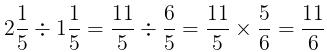Courses

# Test: Fractions and Decimals

## 30 Questions MCQ Test Mathematics (Maths) Class 7 | Test: Fractions and Decimals

Description
This mock test of Test: Fractions and Decimals for Class 7 helps you for every Class 7 entrance exam. This contains 30 Multiple Choice Questions for Class 7 Test: Fractions and Decimals (mcq) to study with solutions a complete question bank. The solved questions answers in this Test: Fractions and Decimals quiz give you a good mix of easy questions and tough questions. Class 7 students definitely take this Test: Fractions and Decimals exercise for a better result in the exam. You can find other Test: Fractions and Decimals extra questions, long questions & short questions for Class 7 on EduRev as well by searching above.
QUESTION: 1

### What is the fraction of the shaded area?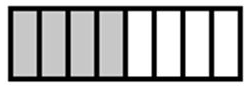Solution:

► Total number of blocks = 8
► Number of shaded blocks = 4
► Number of unshaded blocks = 4

⇒ Fraction of Shaded area =  No. of blocks of shaded area / Total number of blocks = 4/8 = 1/2

QUESTION: 2

### What is the fraction of the shaded area?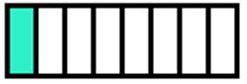Solution:

► Total number of blocks = 8
► Number of shaded blocks = 1
► Number of unshaded blocks = 7

⇒ Fraction of Shaded area =  No. of shaded blocks/ Total number of blocks = 1/8

QUESTION: 3

### What is the fraction of the shaded area?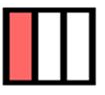Solution:

► Total number of blocks = 3
► Number of shaded blocks = 1
► Number of unshaded blocks = 2

Fraction of Shaded area =  No. of shaded blocks/ Total number of blocks = 1/3

QUESTION: 4

What is the fraction of the shaded area?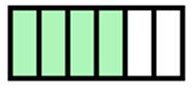Solution:

► Total number of blocks = 6
► Number of shaded blocks = 4
► Number of unshaded blocks = 2

Fraction of Shaded area =  No. of shaded blocks/ Total number of blocks = 4/6 = 2/3

QUESTION: 5

What is the fraction of the shaded area ?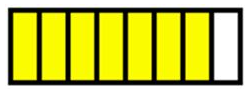Solution:

► Total number of blocks = 8
► Number of shaded blocks = 7
► Number of unshaded blocks = 1

Fraction of Shaded area =  No. of shaded blocks/ Total number of blocks = 7/8

QUESTION: 6

When 0.232323..... is converted into a fraction, then the result is:

Solution:

0.23232323…=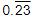Let, x =100x = 23.232323...
⇒ 100x - x = 23.232323...... - 0.23232323......
⇒ 99x = 23
⇒ x = 23/99

QUESTION: 7

Three times the first of three consecutive odd integers is 3 more than twice the third. The third integer is:

Solution:

Let the three integers be x, x + 2 and x + 4.

Then, 3x = 2(x + 4) + 3  ⇔  x = 11.

∴ Third integer = x + 4 = 15.

QUESTION: 8

(1/2) x (1/5) = ____.

Solution:

► (1/2) x (1/5) = 1/10

QUESTION: 9

If 2994 ÷ 14.5 = 172, then 29.94 ÷ 1.45 = ?

Solution:

Given: 2994 ÷ 14.5 = 172

29.94 ÷ 1.45 = 2994 ÷ 145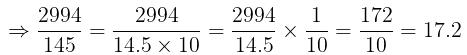QUESTION: 10

0.01 × 0.01 = ______.

Solution:

► 0.01 × 0.01 = 0.0001

QUESTION: 11

Arrange the following in descending order:

(2/9), (2/3), (8/21)

Solution:

First, we convert each fraction into like fraction.

► 2/9 = (2 x 7)/(9 x 7) = 14/63
► 2/3 = (2 x 21)/(3 x 21) = 42/63
► 8/21 = (8 x 2)/(21 x 3) = 24/63.

If denominators are the same, then, greater the numerator, the greater will be the fraction.

∵ 42 > 24 > 14
⇒ (2/3) > (8/21) > (2/9)

Thus, option A is correct.

QUESTION: 12

Ritu ate 3/5 part of an apple and the remaining apple was eaten by her brother Somu. How much part of the apple did Somu eat?

Solution:

► The part of the apple eaten by Ritu = 3/5

► The part of the apple eaten by Somu = 1 - (3/5) = (5 - 3)/5 = 2/5.

QUESTION: 13

One side of a square is 2/a, its perimeter is:

Solution:

► Side of square = 2/a
⇒ Perimeter = 4 x side of square = 4 x 2/a = 8/a

QUESTION: 14

What will be the average of  0.3, 3, 0.03 and 0.002?

Solution:

Given numbers are: 0.3 , 3 , 0.03 and 0.002
► Average =  Sum of numbers divided by total numbers

⇒ Average = (0.3 + 3 + 0.03 + 0.002)/4 = 3.332 / 4 = 0.833

QUESTION: 15

The result of adding the difference of 3.003 and 2.05 to their sum is:

Solution:

Given numbers are: 3.003 and 2.05

► Sum of numbers = 3.003 + 2.05 = 5.053
► Difference of numbers = 3.003 - 2.05  = 0.953

Adding the Difference of numbers to the Sum of numbers, 5.053 + 0.953 = 6.006

QUESTION: 16

Find the area of rectangle whose length is 6.7 cm and breadth is 2 cm.

Solution:

Given: Length, L = 6.7 cm, Breadth, B = 2 cm

► Area of rectangle = L x B = 6.7 x 2 = 13.4 cm2

QUESTION: 17

If 43m = 0.086 then m has the value:

Solution:

43m = 0.086
⇒ m = 0.086/43 = 0.002

QUESTION: 18

The value of  2.5 × 0.3 is:

Solution:

► 2.5 × 0.3 = 0.75

QUESTION: 19

The fraction (p+q)/q equals:

Solution:

► (p+q)/q = p/q + q/q = p/q + 1

QUESTION: 20

What is 3/5 of 25?

Solution:

► (3/5) of 25=  (3/5) x 25 = 3 x 5 = 15

QUESTION: 21

5/7 ÷ 1/2 is equal to?

Solution:

► (5/7) ÷ (1/2) = (5/7) x (2/1) = 10/7

QUESTION: 22

Which of the following is an improper fraction?

Solution:

A fraction in which the numerator is greater than the denominator is called an improper fraction.

Option A: 20 is less than 70
Option B: 30 is less than 40
Option C: 50 is greater than 20
Option D: 70 is less than 80

Thus, option C is the correct.

QUESTION: 23

Which of the following is an equivalent fraction of  3/10?

Solution:

Two fractions are said to be equivalent fractions if the simplest form of both the fractions is the same.

Option A: 6/20 = 3/10
Option B: 5/20 = 1/4
Option C: 1/40 = 1/40

Thus option A is correct.

QUESTION: 24

2/3 of 66 is:

Solution:

► (2/3) of 66 = (2/3) x 66 = 44

QUESTION: 25

The product of two proper fractions is ______ than each of the fractions that are multiplied

Solution:

The product of two proper fractions is less than each of the fractions that are multiplied.
Example: 1/2 x 1/4 = 1/8
1/8 < 1/2 and 1/8 < 1/4

QUESTION: 26

Which two fractions are equivalent?

Solution:

► Divide whole of the fraction 2/4 by 2, we get 1/2.

QUESTION: 27

Express as rupees using decimals: 7 rupees 7 paise.

Solution:

► 7 rupees 7 paise = Rs. 7 + Rs. (7/100) = Rs. 7 + Rs. 0.07 = Rs. 7.07

QUESTION: 28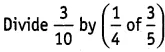Solution: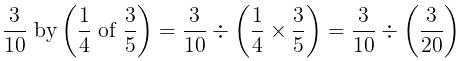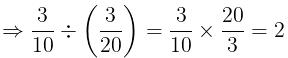QUESTION: 29

2.05 x 1.3 equals to?

Solution:

► 2.05 x 1.3 = 2.665

QUESTION: 30

What will be the value of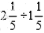?

Solution: